# The Dot Game

The Dot Game
Math - Arithmetical Operations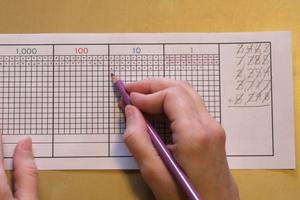LevelPrimary
Age5+
Multiplication With the Stamp Game
Materials Dot Game Paper
pencil
purple or orange pencil

This activity introduces the child to the 10,000 place value and allows the child a further level of mathematical abstraction in addition and multiplication.
Two versions of printable Dot Game Paper are available below.

## Presentation

1. Take the material to a table (could also be done on a clipboard).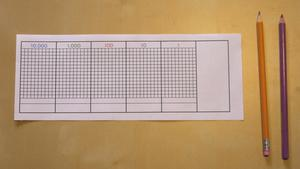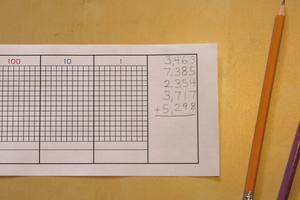3. Point out the units in the first number.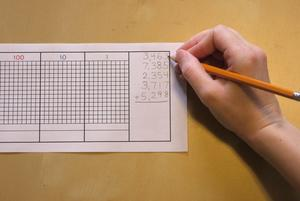4. Starting from the top left, put dots in the first spaces in the units section.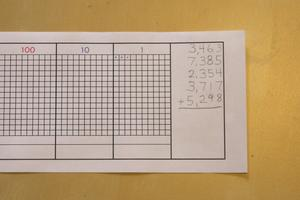5. Put a line through the units in the first number.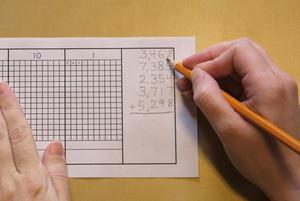6. Do the same with the tens...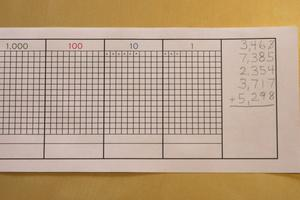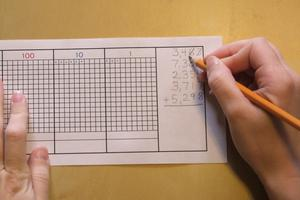the hundreds...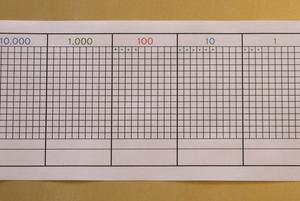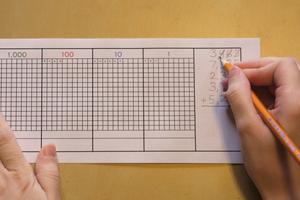and the thousands.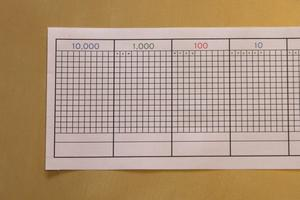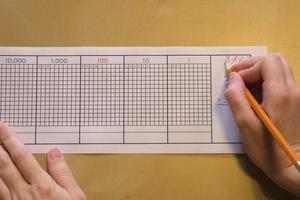7. Point out the units in the second number.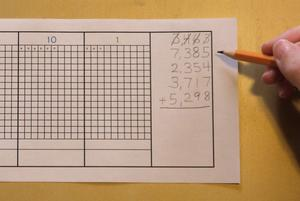8. Start filling in dots in the next available units space.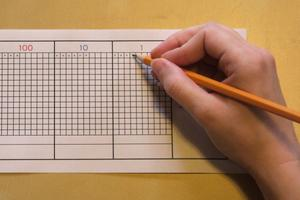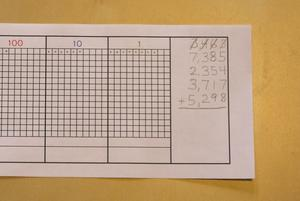9. Then cross out the number.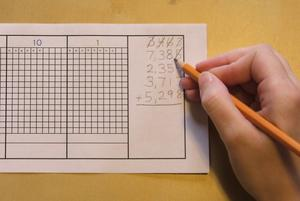10. Fill in the dots for rest of the numbers in the same way.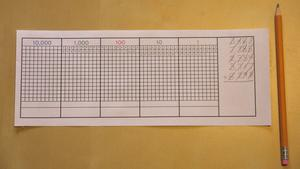11. Switch to the purple pencil now.
Count to see that each row has ten dots in it. Cross off a row...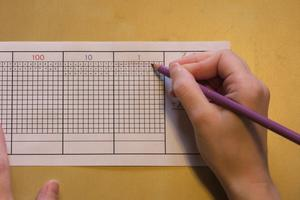12. and add a purple dot to the open section below.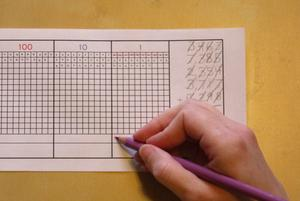13. Do the same with any other full rows of dots.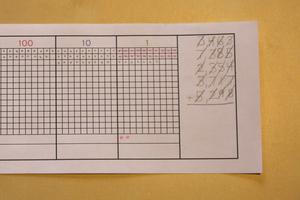14. Point out the dots that are left that do not make up a full row.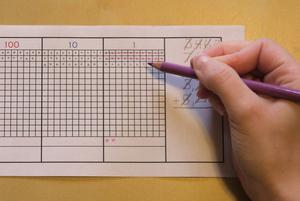15. Record that number in the bottom space.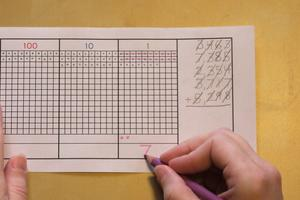16. Point out the purple dots indicating the the full rows of ten units each.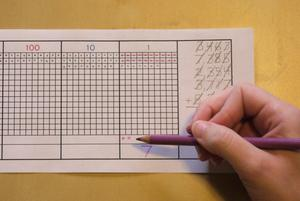17. Add the same number of purple dots to the tens section.18. Cross off groups of 10 tens as was done with the units. Record the remaining tens at the bottom.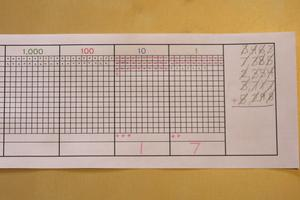19. Do the same with the hundreds...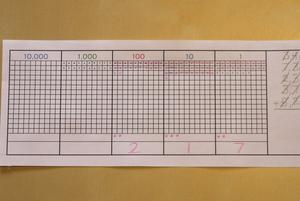and the thousands.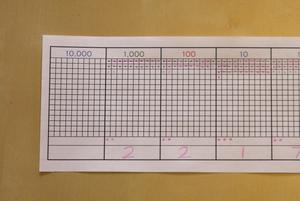20. Move the dots from the thousands to the ten-thousands section.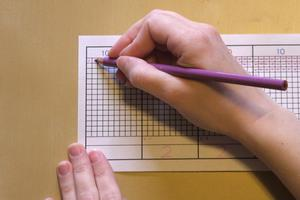21. Record the number of ten-thousands in the bottom space.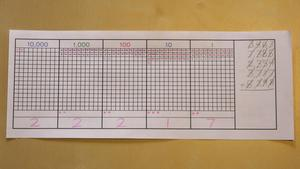22. Write the answer under the original problem.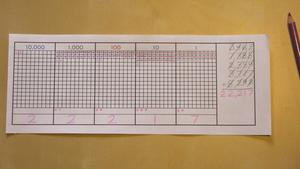## Control of Error

Teacher subsequently the children

## Material

It's amazing how much some of the suppliers charge for dot game paper. I don't know about you, but I think that 66¢ for a sheet of paper that's going to be used for one math problem is a bit high. Just print your own from one of the PDFs below. You could even laminate a sheet and use it with dry erase markers.
Printables:

Dry Erase Dot Game Board
Albanesi \$57.00
Alison's \$20.00
Alison's \$50.00